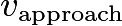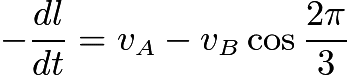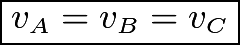# Really interesting and tough kinematics problem

PhysicsKT

## Homework Statement

Three ants A, B and C are crawling on a large horizontal tabletop always occupying vertices of an equilateral triangle, size of which may vary with time. If at an instant, speeds of A and B are vA and vB, prove that vC>= vA+vB.

## Homework Equations

Well, that's what I don't understand- to always maintain the shape of an equilateral triangle, one of the paths they may acquire is constantly approach each other.

## The Attempt at a Solution

First I considered them to move along the equilateral triangle- but using cosine law doesn't help. If they approach each other, I got the relation that their velocities must be equal since:

We write thefor A approaching B as. Now, all the velocity of approaches must be equal in order to form an equilateral triangle at every point. Soare all equal, which means thatThis gives that.

What to do now in order to make a solid conclusion?

Homework Helper
Gold Member
2022 Award

## Homework Statement

Three ants A, B and C are crawling on a large horizontal tabletop always occupying vertices of an equilateral triangle, size of which may vary with time. If at an instant, speeds of A and B are vA and vB, prove that vC>= vA+vB.

## Homework Equations

Well, that's what I don't understand- to always maintain the shape of an equilateral triangle, one of the paths they may acquire is constantly approach each other.

## The Attempt at a Solution

First I considered them to move along the equilateral triangle- but using cosine law doesn't help. If they approach each other, I got the relation that their velocities must be equal since:

We write the View attachment 236385 for A approaching B as View attachment 236386. Now, all the velocity of approaches must be equal in order to form an equilateral triangle at every point. So View attachment 236387 are all equal, which means thatView attachment 236388This gives that View attachment 236389.

What to do now in order to make a solid conclusion?
I assume you mean |vC|≤|vA|+|vB|.
I think you have a sign error in your dl/dt equation, but anyway the approach is not general enough. You need to prove it is always true.
Try considering a frame of reference that simplifies matters.

PhysicsKT
I meant |vC| =< |vA|+|vB|

My -dl/dt equation is correct.

Also, to go into one's reference frame, we actually need to know its path first, which we don't. Any useful hints on its path?
I agree my approach is not general enough- so suggest me one please.

Last edited:
Staff Emeritus
Homework Helper
Gold Member
I meant |vC| >= |vA|+|vB|
This is quite obviously false as it is easy to construct a counter example.

•CWatters and PhysicsKT
Homework Helper
Gold Member
2022 Award
My -dl/dt equation is correct.
Yes, I did not notice you had 2π/3 inside the cos. I was expecting π/3.
to go into one's reference frame
Not one ant as the reference frame, something more symmetrical.
How might you parameterise the motion of an equilateral triangle within its plane, allowing for varying size?

PhysicsKT
This is quite obviously false as it is easy to construct a counter example.
OOPS sorry for the typo.

PhysicsKT
Yes, I did not notice you had 2π/3 inside the cos. I was expecting π/3.

Not one ant as the reference frame, something more symmetrical.
How might you parameterise the motion of an equilateral triangle within its plane, allowing for varying size?
I don't know, please guide me how to parameterise the motion.

Homework Helper
Gold Member
2022 Award
I don't know, please guide me how to parameterise the motion.
I recommend to use complex variables here to represent the positions of the corners.
If the centre is at z and one vertex is at z+re, where are the other two vertices?

•PhysicsKT and PeroK
Homework Helper
Gold Member
2022 Award
I don't know, please guide me how to parameterise the motion.

What about using a frame of reference in which one vertex is at rest?

Homework Helper
Gold Member
2022 Award
What about using a frame of reference in which one vertex is at rest?
I think that's rather messier than my approach in post #8. Have you tried your way?
Remember that it will not suffice to show it is true in one particular reference frame.

Homework Helper
Gold Member
2022 Award
I think that's rather messier than my approach in post #8. Have you tried your way?
Remember that it will not suffice to show it is true in one particular reference frame.

Your complex variables approach looks better.

J Hann
One way might be to place the center of the triangle at the origin and orient the triangle such that
the instantaneous velocity of Vc is along the y-axis.
Then resolve the velocities into their x-y components.
Note that then VBy = VAy, VCx = 0, and VAx = - VBx at that instant in time
if the triangle is to remain an equilateral triangle.
You also have the relation that VAx = VBy tan 30.

•PhysicsKT
Homework Helper
Gold Member
2022 Award
One way might be to place the center of the triangle at the origin and orient the triangle such that
the instantaneous velocity of Vc is along the y-axis.
Then resolve the velocities into their x-y components.
Note that then VBy = VAy, VCx = 0, and VAx = - VBx at that instant in time
if the triangle is to remain an equilateral triangle.
You also have the relation that VAx = VBy tan 30.
But as I noted, it is not sufficient to demonstrate the result within a chosen reference frame. It has to be shown to be true in the lab frame.

PhysicsKT
I recommend to use complex variables here to represent the positions of the corners.
If the centre is at z and one vertex is at z+re, where are the other two vertices?
I'm not very comfortable using complex numbers- can you write down the whole solution?

Homework Helper
Gold Member
2022 Award
I'm not very comfortable using complex numbers- can you write down the whole solution?
That's not how things work on these homework forums. (You should not even ask!)
Can you make an attempt at the question I posed in post #8?

By the way, I am not at all sure I have an efficient solution. It is still a lot of work. There might be something much neater.

•PeroK
Homework Helper
Gold Member
2022 Award
Just an idea. In the reference frame of centre of the triangle the three velocity vectors form an equilateral triangle.

All you have to consider then is adding the velocity of the centre.

Homework Helper
Gold Member
I stumbled on a way to get the answer without too much work. It's based on a particular way of expressing the position of C in terms of the positions of A and B.

Let the position vectors of the three ants be ##\vec R_A##, ##\vec R_B##, and ##\vec R_C## relative to an arbitrarily chosen origin. Also, let ##\hat k## be a unit vector perpendicular to the tabletop.

@PhysicsKT , which of the following is correct?

(a) ##\vec R_C = \frac{1}{2} \left(\vec R_A + \vec R_B \right) + \frac{1}{2} \left(\vec R_A - \vec R_B \right)\times \hat k ##

(b) ##\vec R_C = \frac{1}{2} \left(\vec R_A + \vec R_B \right) + \frac{\sqrt 3}{2} \left(\vec R_A - \vec R_B \right)\times \hat k ##

(c) ##\vec R_C = \frac{1}{2} \left(\vec R_A - \vec R_B \right) + \frac{1}{2} \left(\vec R_A + \vec R_B \right)\times \hat k ##

(d) ##\vec R_C = \frac{1}{2} \left(\vec R_A - \vec R_B \right) + \frac{\sqrt 3}{2} \left(\vec R_A + \vec R_B \right)\times \hat k ##

Use the correct choice to express the velocity ##\vec V_C## in terms of the velocities ##\vec V_A## and ##\vec V_B##.

Last edited:
•PhysicsKT, Delta2 and PeroK
Homework Helper
Gold Member
2022 Award
Using the idea in post #16 The problem reduces to showing that for an equilareral triangle the distance of any vertex from the origin is always less than the sum of the distances from the origin to the other two vertices. And, in fact, you can show the stronger condition that:

##|OA|^2 \le |OB|^2 + |OC|^2##

This looks fairly obvious and can be shown with a little algebra.

•PhysicsKT
Homework Helper
Gold Member
2022 Award
Using the idea in post #16 The problem reduces to showing that for an equilareral triangle the distance of any vertex from the origin is always less than the sum of the distances from the origin to the other two vertices. And, in fact, you can show the stronger condition that:

##|OA|^2 \le |OB|^2 + |OC|^2##

This looks fairly obvious and can be shown with a little algebra.
You are still overlooking that it does not suffice to obtain the result in one chosen reference frame. One needs to show it is true regardless of the way the triangle as a whole moves, rotates and grows/shrinks.

•PhysicsKT
Homework Helper
Gold Member
2022 Award
I stumbled on a way to get the answer without too much work. It's based on a particular way of expressing the position of C in terms of the positions of A and B.

Let the position vectors of the three ants be ##\vec R_A##, ##\vec R_B##, and ##\vec R_C## relative to an arbitrarily chosen origin. Also, let ##\hat k## be a unit vector perpendicular to the tabletop.

@PhysicsKT , which of the following is correct?

(a) ##\vec R_C = \frac{1}{2} \left(\vec R_A + \vec R_B \right) + \frac{1}{2} \left(\vec R_A - \vec R_B \right)\times \hat k ##

(b) ##\vec R_C = \frac{1}{2} \left(\vec R_A + \vec R_B \right) + \frac{\sqrt 3}{2} \left(\vec R_A - \vec R_B \right)\times \hat k ##

(c) ##\vec R_C = \frac{1}{2} \left(\vec R_A - \vec R_B \right) + \frac{1}{2} \left(\vec R_A + \vec R_B \right)\times \hat k ##

(d) ##\vec R_C = \frac{1}{2} \left(\vec R_A - \vec R_B \right) + \frac{\sqrt 3}{2} \left(\vec R_A + \vec R_B \right)\times \hat k ##

Use the correct choice to express the velocity ##\vec V_C## in terms of the velocities ##\vec V_A## and ##\vec V_B##.
Yes, this is the vector equivalent of the path I was taking with complex variables. Not sure if one turns out easier than the other... I would expect it is the same.

•PhysicsKT and TSny
Homework Helper
Gold Member
Yes, this is the vector equivalent of the path I was taking with complex variables. Not sure if one turns out easier than the other... I would expect it is the same.
Yes. The cross product with ##\hat k## in the vector approach corresponds to multiplication by ##i## in the complex variable approach. I actually like the complex variable method better. The expressions are cleaner. But the OP wasn't comfortable with it.

Surprising to me, with either approach, not much calculation is required to get the desired result.

Last edited:
Homework Helper
Gold Member
2022 Award
You are still overlooking that it does not suffice to obtain the result in one chosen reference frame. One needs to show it is true regardless of the way the triangle as a whole moves, rotates and grows/shrinks.

Of course I'm not overlooking that. In the rest frame of the centre of the triangle the velocity vectors of the vertices form an equilateral triangle. Adding the arbitrary velocity of the centre gives an equilateral triangle anywhere in the plane.

The problem, therefore, reduces to showing the position vectors of the vertices of any equilateral triangle obey the required inequality.

•haruspex
Homework Helper
Gold Member
2022 Award
Of course I'm not overlooking that. In the rest frame of the centre of the triangle the velocity vectors of the vertices form an equilateral triangle. Adding the arbitrary velocity of the centre gives an equilateral triangle anywhere in the plane.

The problem, therefore, reduces to showing the position vectors of the vertices of any equilateral triangle obey the required inequality.
My apologies... I had not fully understood your method. This could be the best way.

Edit: but so far, it looks equivalent to the methods TSny and I have proposed. Showing those vectors satisfy the inequality does not appear trivial. Maybe you have another trick I am missing.

Last edited:
•PeroK
Homework Helper
Gold Member
And, in fact, you can show the stronger condition that:

##|OA|^2 \le |OB|^2 + |OC|^2##
Is there a restriction on the location of the origin? If not, then I don't think this relation is always true. For example, take the origin O to be the midpoint between B and C.

Anyway, I'm still trying to work out your approach. So far, no luck. But I will keep thinking about it. Fun problem.

•PeroK
Staff Emeritus
Homework Helper
Gold Member
Is there a restriction on the location of the origin? If not, then I don't think this relation is always true. For example, take the origin O to be the midpoint between B and C.

Anyway, I'm still trying to work out your approach. So far, no luck. But I will keep thinking about it. Fun problem.
I'm pretty sure that @PeroK 's origin is at the center of the triangle.

Homework Helper
Gold Member
I'm pretty sure that @PeroK 's origin is at the center of the triangle.
OK, thanks. I had noticed in post #16 that @PeroK might be taking the center of the triangle as the origin. But, if so, then ##|OA| = |OB| = |OC|## and the following statement would truly be obvious.
... you can show the stronger condition that:

##|OA|^2 \le |OB|^2 + |OC|^2##

This looks fairly obvious and can be shown with a little algebra.

Homework Helper
Gold Member
2022 Award
I'm pretty sure that @PeroK 's origin is at the center of the triangle.
No, it is not. See post #22.

Fun fact: equality is achieved if the origin is anywhere on the circle through the vertices of the triangle.

Last edited:
•SammyS and PeroK